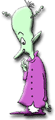Math Fundamentals Percent Conversions to Fractions & Decimals1. Write 25% as a decimal. 2. Write 6.75 as a percent. 3. Write 78% as a fraction. 4. Write 98.5% as a decimal. 5. Write 0.5 % as a decimal. 6.Write 0.006 as a fraction 7. Write 80% as a fraction. 8. Write 0.23 as a percent 9. Write 200% as a decimal or whole number. 10. Write 0.006 as a percent. 11. Write 22% as a fraction. 12. Write 3.5% as a decimal. 13. Write 3/4 as a percent. 14.Write 4/5 as a percent. 15. Write 7/10 as a percent.All Math Contents Copyright©1997 - 2014 by Ellen Freedman, All Rights Reserved.